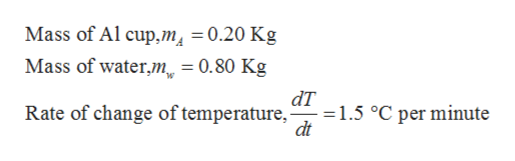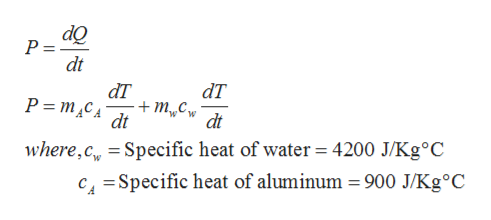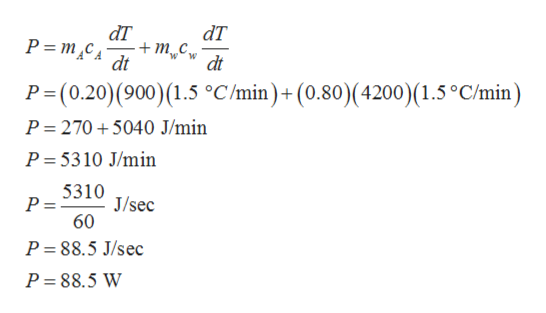# A 0.200-kg aluminum cup contains 800. g of water in thermal equilibrium with the cup at 80.°C. The combination of cup and water is cooled uniformly so that the temperature decreases by 1.5°C per minute. At what rate is energy being removed? Express your answer in watts.

Question
25 views

A 0.200-kg aluminum cup contains 800. g of water in thermal equilibrium with the cup at 80.°C. The combination of cup and water is cooled uniformly so that the temperature decreases by 1.5°C per minute. At what rate is energy being removed? Express your answer in watts.

check_circle

Step 1

Given,help_outlineImage TranscriptioncloseMass of Al cup,m̟ =0.20 Kg Mass of water,m = 0.80 Kg dT =1.5 °C per minute dt Rate of change of temperature, fullscreen
Step 2

Rate at which energy is removed is known as Power. Rate of removal of energy can be calculated as,help_outlineImage TranscriptionclosedQ dt dT đT +m„Cw dt Р -тса dt where,c, = Specific heat of water = 4200 J/KG°C c = Specific heat of aluminum = 900 J/Kg°C fullscreen
Step 3

By plugging in the g...help_outlineImage TranscriptionclosedT + т.с, dt đT P= m,C4 dt P=(0.20)(900)(1.5 °C/min)+(0.80)(4200)(1.5°C/min) P= 270 + 5040 J/min P = 5310 J/min 5310 P =S10 J/sec 60 P = 88.5 J/sec P = 88.5 W fullscreen

### Want to see the full answer?

See Solution

#### Want to see this answer and more?

Solutions are written by subject experts who are available 24/7. Questions are typically answered within 1 hour.*

See Solution
*Response times may vary by subject and question.
Tagged in

### Heat Transfer